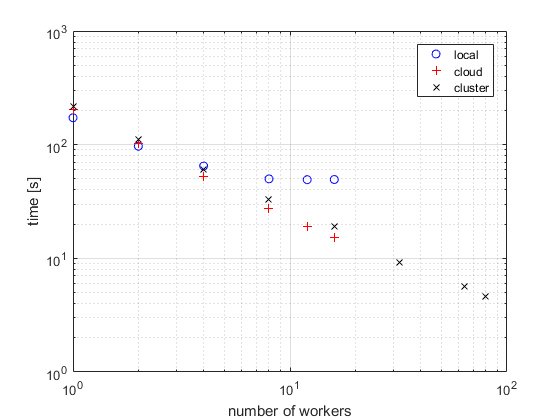## Scale Up `parfor`-Loops to Cluster and Cloud

In this example, you start on your local multicore desktop and measure the time required to run a calculation, as a function of increasing numbers of workers. The test is called a strong scaling test. It enables you to measure the decrease in time required for the calculation if you add more workers. This dependence is known as speedup, and allows you to estimate the parallel scalability of your code. You can then decide whether it is useful to increase the number of workers in your parallel pool, and scale up to cluster and cloud computing.

1. Create the function.

` edit MyCode`
2. In the MATLAB® Editor, enter the new `parfor`-loop and add `tic` and `toc` to measure the time elapsed.

```function a = MyCode(A) tic parfor i = 1:200 a(i) = max(abs(eig(rand(A)))); end toc ```
3. Save the file, and close the Editor.

4. On the Parallel > Parallel Preferences menu, check that your Default Cluster is local (your desktop machine).

5. In the MATLAB Command Window, define a parallel pool of size 1, and run your function on one worker to calculate the elapsed time. Note the elapsed time for a single worker and shut down your parallel pool.

```parpool(1); a = MyCode(1000); ```
```Elapsed time is 172.529228 seconds. ```
```delete(gcp); ```
6. Open a new parallel pool of two workers, and run the function again.

```parpool(2); a = MyCode(1000); ```

Note the elapsed time; you should see that this now has decreased compared to the single worker case.

7. Try 4, 8, 12 and 16 workers. Measure the parallel scalability by plotting the elapsed time for each number of workers on a log-log scale.The figure shows the scalability for a typical multicore desktop PC (blue circle data points). The strong scaling test shows almost linear speedup and significant parallel scalability for up to eight workers. Observe from the figure that, in this case, we do not achieve further speedup for more than eight workers. This result means that, on a local desktop machine, all cores are fully used for 8 workers. You can get a different result on your local desktop, depending on your hardware. To further speed up your parallel application, consider scaling up to cloud or cluster computing.

8. If you have exhausted your local workers, as in the previous example, you can scale up your calculation to cloud computing. Check your access to cloud computing from the Parallel > Discover Clusters menu.

Open a parallel pool in the cloud and run your application without changing your code.

```parpool(16); a = MyCode(1000); ```

Note the elapsed time for increasing numbers of cluster workers. Measure the parallel scalability by plotting the elapsed time as a function of number of workers on a log-log scale.The figure shows typical performance for workers in the cloud (red plus data points). This strong scaling test shows linear speedup and 100% parallel scalability up to 16 workers in the cloud. Consider further scaling up of your calculation by increasing the number of workers in the cloud or on a compute cluster. Note that the parallel scalability can be different, depending on your hardware, for a larger number of workers and other applications.

9. If you have direct access to a cluster, you can scale up your calculation using workers on the cluster. Check your access to clusters from the Parallel > Discover Clusters menu. If you have an account, select cluster, open a parallel pool and run your application without changing your code.

```parpool(64); a = MyCode(1000); ```The figure shows typical strong scaling performance for workers on a cluster (black x data points). Observe that you achieve 100% parallel scalability, persisting up to at least 80 workers on the cluster. Note that this application scales linearly - the speedup is equal to the number of workers used.

This example shows a speedup equal to the number of workers. Not every task can achieve a similar speedup, see for example Interactively Run a Loop in Parallel Using parfor.

### Tip

You can further profile a `parfor`-loop by measuring how much data is transferred to and from the workers in the parallel pool by using `ticBytes` and `tocBytes`. For more information and examples, see Profiling parfor-loops.

## Related Topics

##### SupportGet trial now